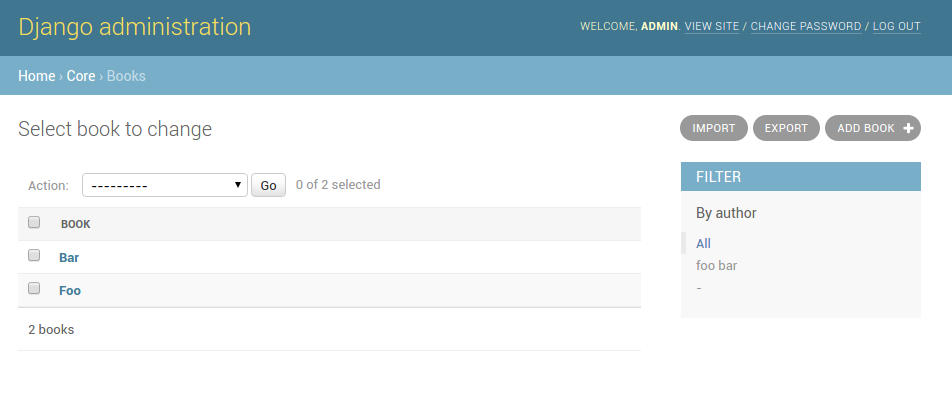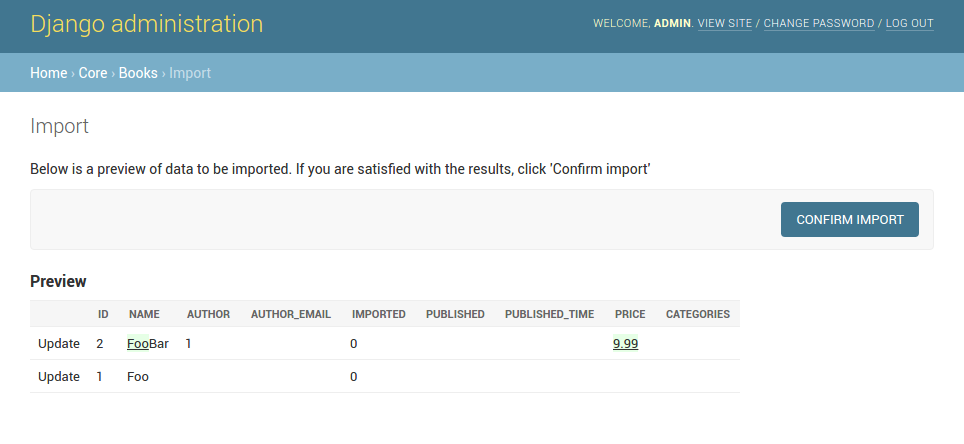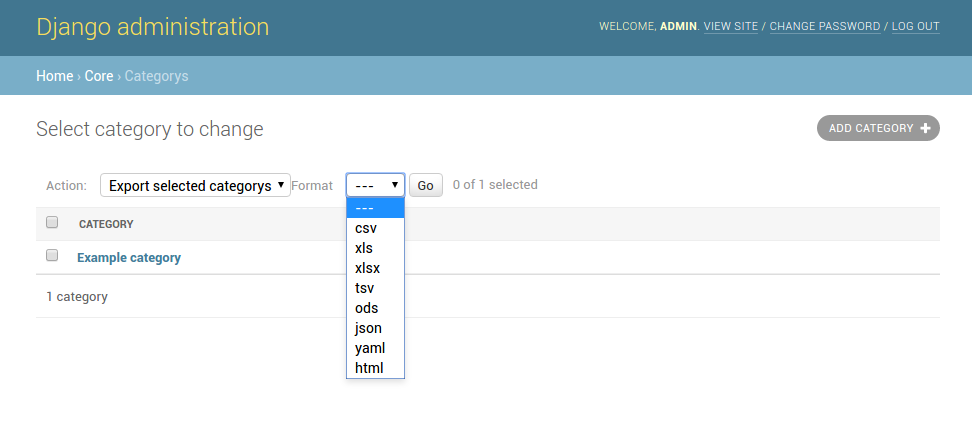# Getting started¶

For example purposes, we’ll use a simplified book app. Here is our `models.py`:

```# app/models.py

class Author(models.Model):
name = models.CharField(max_length=100)

def __unicode__(self):
return self.name

class Category(models.Model):
name = models.CharField(max_length=100)

def __unicode__(self):
return self.name

class Book(models.Model):
name = models.CharField('Book name', max_length=100)
author = models.ForeignKey(Author, blank=True, null=True)
author_email = models.EmailField('Author email', max_length=75, blank=True)
imported = models.BooleanField(default=False)
published = models.DateField('Published', blank=True, null=True)
price = models.DecimalField(max_digits=10, decimal_places=2, null=True, blank=True)
categories = models.ManyToManyField(Category, blank=True)

def __unicode__(self):
return self.name
```

## Creating import-export resource¶

To integrate django-import-export with our `Book` model, we will create a `ModelResource` class in `admin.py` that will describe how this resource can be imported or exported:

```# app/admin.py

from import_export import resources
from core.models import Book

class BookResource(resources.ModelResource):

class Meta:
model = Book
```

## Exporting data¶

Now that we have defined a `ModelResource` class, we can export books:

```>>> from app.admin import BookResource
>>> dataset = BookResource().export()
>>> print dataset.csv
id,name,author,author_email,imported,published,price,categories
2,Some book,1,,0,2012-12-05,8.85,1
```

## Customize resource options¶

By default `ModelResource` introspects model fields and creates `Field`-attributes with an appropriate `Widget` for each field.

To affect which model fields will be included in an import-export resource, use the `fields` option to whitelist fields:

```class BookResource(resources.ModelResource):

class Meta:
model = Book
fields = ('id', 'name', 'price',)
```

Or the `exclude` option to blacklist fields:

```class BookResource(resources.ModelResource):

class Meta:
model = Book
exclude = ('imported', )
```

An explicit order for exporting fields can be set using the `export_order` option:

```class BookResource(resources.ModelResource):

class Meta:
model = Book
fields = ('id', 'name', 'author', 'price',)
export_order = ('id', 'price', 'author', 'name')
```

The default field for object identification is `id`, you can optionally set which fields are used as the `id` when importing:

```class BookResource(resources.ModelResource):

class Meta:
model = Book
import_id_fields = ('isbn',)
fields = ('isbn', 'name', 'author', 'price',)
```

When defining `ModelResource` fields it is possible to follow model relationships:

```class BookResource(resources.ModelResource):

class Meta:
model = Book
fields = ('author__name',)
```

Note

Following relationship fields sets `field` as readonly, meaning this field will be skipped when importing data.

By default all records will be imported, even if no changes are detected. This can be changed setting the `skip_unchanged` option. Also, the `report_skipped` option controls whether skipped records appear in the import `Result` object, and if using the admin whether skipped records will show in the import preview page:

```class BookResource(resources.ModelResource):

class Meta:
model = Book
skip_unchanged = True
report_skipped = False
fields = ('id', 'name', 'price',)
```

Resources

## Declaring fields¶

It is possible to override a resource field to change some of its options:

```from import_export import fields

class BookResource(resources.ModelResource):
published = fields.Field(column_name='published_date')

class Meta:
model = Book
```

Other fields that don’t exist in the target model may be added:

```from import_export import fields

class BookResource(resources.ModelResource):
myfield = fields.Field(column_name='myfield')

class Meta:
model = Book
```

Fields
Available field types and options.

Not all data can be easily extracted from an object/model attribute. In order to turn complicated data model into a (generally simpler) processed data structure, `dehydrate_<fieldname>` method should be defined:

```from import_export import fields

class BookResource(resources.ModelResource):
full_title = fields.Field()

class Meta:
model = Book

def dehydrate_full_title(self, book):
return '%s by %s' % (book.name, book.author.name)
```

## Customize widgets¶

A `ModelResource` creates a field with a default widget for a given field type. If the widget should be initialized with different arguments, set the `widgets` dict.

In this example widget, the `published` field is overriden to use a different date format. This format will be used both for importing and exporting resource.

```class BookResource(resources.ModelResource):

class Meta:
model = Book
widgets = {
'published': {'format': '%d.%m.%Y'},
}
```

Widgets
available widget types and options.

## Importing data¶

Let’s import some data!

 ```1 2 3 4 5 6 7 8 9``` ```>>> import tablib >>> from import_export import resources >>> from core.models import Book >>> book_resource = resources.modelresource_factory(model=Book)() >>> dataset = tablib.Dataset(['', 'New book'], headers=['id', 'name']) >>> result = book_resource.import_data(dataset, dry_run=True) >>> print result.has_errors() False >>> result = book_resource.import_data(dataset, dry_run=False) ```

In the fourth line we use `modelresource_factory()` to create a default `ModelResource`. The ModelResource class created this way is equal to the one shown in the example in section Creating import-export resource.

In fifth line a `Dataset` with columns `id` and `name`, and one book entry, are created. A field for a primary key field (in this case, `id`) always needs to be present.

In the rest of the code we first pretend to import data using `import_data()` and `dry_run` set, then check for any errors and actually import data this time.

Import data workflow
for a detailed description of the import workflow and its customization options.

### Deleting data¶

To delete objects during import, implement the `for_delete()` method on your `Resource` class.

The following is an example resource which expects a `delete` field in the dataset. An import using this resource will delete model instances for rows that have their column `delete` set to `1`:

```class BookResource(resources.ModelResource):
delete = fields.Field(widget=widgets.BooleanWidget())

def for_delete(self, row, instance):
return self.fields['delete'].clean(row)

class Meta:
model = Book
```

## Signals¶

To hook in the import export workflow, you can connect to `post_import`, `post_export` signals:

```from django.dispatch import receiver
from import_export.signals import post_import, post_export

def _post_import(model, **kwargs):
# model is the actual model instance which after import
pass

def _post_export(model, **kwargs):
# model is the actual model instance which after export
pass
```

### Exporting via list filters¶

Admin integration is achieved by subclassing `ImportExportModelAdmin` or one of the available mixins (`ImportMixin`, `ExportMixin`, `ImportExportMixin`):

```# app/admin.py

resource_class = BookResource
```A screenshot of the change view with Import and Export buttons.A screenshot of the import view.A screenshot of the confirm import view.

Another approach to exporting data is by subclassing `ImportExportActionModelAdmin` which implements export as an admin action. As a result it’s possible to export a list of objects selected on the change list page:

```# app/admin.py